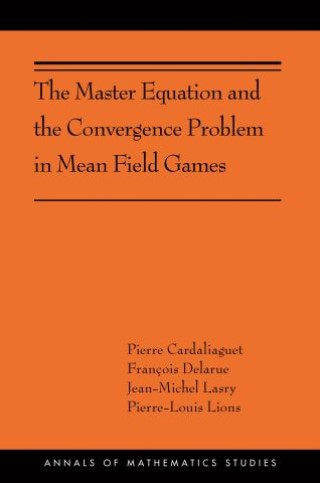## The Master Equation and the Convergence Problem in Mean Field Games• Description
• Author
• Info
• Reviews

### Description

This book describes the latest advances in the theory of mean field games, which are optimal control problems with a continuum of players, each of them interacting with the whole statistical distribution of a population. While it originated in economics, this theory now has applications in areas as diverse as mathematical finance, crowd phenomena, epidemiology, and cybersecurity.

Because mean field games concern the interactions of infinitely many players in an optimal control framework, one expects them to appear as the limit for Nash equilibria of differential games with finitely many players as the number of players tends to infinity. This book rigorously establishes this convergence, which has been an open problem until now. The limit of the system associated with differential games with finitely many players is described by the so-called master equation, a nonlocal transport equation in the space of measures. After defining a suitable notion of differentiability in the space of measures, the authors provide a complete self-contained analysis of the master equation. Their analysis includes the case of common noise problems in which all the players are affected by a common Brownian motion. They then go on to explain how to use the master equation to prove the mean field limit.

This groundbreaking book presents two important new results in mean field games that contribute to a unified theoretical framework for this exciting and fast-developing area of mathematics.